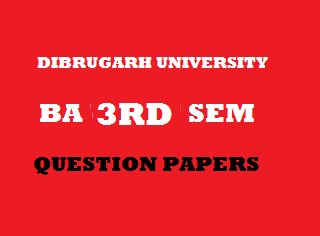## Thursday, January 03, 20192013
(November)
ECONOMICS
(Major)
Course: 301
(Microeconomics - II)
Full Marks: 80
Pass Marks: 32
Time: 3 hours
The figures in the margin indicate full marks for the questions

1. Choose and write the correct one of the following: 1x8=8
1. If the firms under perfect competition have different costs, normal profit will be earned by
1. The marginal firm.
2. The intramarginal firm.
3. All the firms.
4. None of the above.
1. In perfectly competitive market
1. Firm is the price giver and industry is the price taker.
2. Firm is the price taker and industry is the price giver.
3. Both are price takers.
4. None of the above.
1. Price discrimination is possible if two markets have
1. Rising cost curves.
2. Declining cost curves.
3. Different elasticity of demand.
4. Equal elasticity of demand.
1. Which of the following is a characteristic of monopolistic competition?
1. Large number of firms and homogeneous product.
2. Single firm and differentiated product.
3. Large number of firms and differentiated product.
4. Only a few firms and similar product.
1. The slope of the MR curve in monopoly market is ____ that of the AR curve.
1. Twice.
2. Half.
3. One-third.
4. Thrice.
1. Monopolistic competition and oligopoly are alike in terms of
1. Non-price competition.
2. Strong mutual interdependence among firms.
3. Kinked demand analysis.
4. The number of firms.
1. Economic rent is earned by a factor when its
1. Supply is perfectly elastic.
2. Supply is inelastic.
3. Supply is more than its demand.
4. None of the above.
1. Externality refers to
1. External economies.
2. External diseconomies.
3. Both (i) and (ii).
4. Neither (i) nor (ii).
2. Write short notes on any four of the following: (within 150 words each): 4x4=16
1. Normal profit.
2. Monopsony.
3. Selling cost.
5. The efficiency of competitive market.
6. Quasi-rent.
3. (a) Show graphically how long-run supply curves of an industry are derived in perfect competition under different cost conditions. 11
Or
(b) What is meant by equilibrium of a firm? Analyze the essential difference in the nature of equilibrium of a firm under perfect competition in the short run and in the long run with diagrams. 2+9=11
4. (a) Compare the equilibrium price and output of a monopolist and a perfectly competitive firm. 11
Or
(b) Why should Government control monopoly price? Under what condition is monopoly economically desirable? Explain the criteria for price control. 2+2+7=11
5. (a) Illustrate how a firm in monopolistic competition reaches its equilibrium in the short run. Does a firm in equilibrium in monopolistic competition always make supernormal profit? 9+2=11
Or
(b) Mention the properties of monopolistic competition. Can monopolistic competition ensure economic efficiency? Justify your answer. 3+8=11
6. (a) Explain the process of wage rate determination under perfectly competitive and imperfectly competitive factor markets. 6+6=12
Or
(b) Explain Ricardo’s theory of rent. Explain the modifications made by the modern theory over the Ricardo’s theory of rent. 6+6=12
7. (a) What is general equilibrium? Illustrate the interrelationship and interdependence of markets.    3+8=11
Or

(b) Analyze the concepts of efficiency in exchange and efficiency in production. What is market failure? State the factors that cause market failure. 6+2+3=11